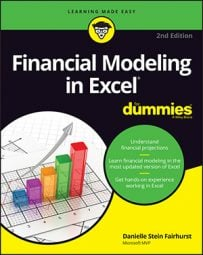##### Financial Modeling in Excel For DummiesOne tool that’s very useful for financial modeling is goal seek. If you know the answer you want, but you want to know what input you need to achieve it, you can work backward using a goal seek.

In order to run a goal seek, you must have

• A formula
• A hard coded input cell that drives this formula
It doesn’t matter how complicated the model is. As long as there is a direct link between the formula and the input cell, the goal seek will calculate the result correctly.

The input cell must be hard coded. It won’t work if the input cell contains a formula.

## Limiting project costs with a goal seek

What a goal seek is and how it works is best demonstrated using a simple model. For a practical example of how to use a goal seek to limit project costings, follow this series of steps as shown.

Let’s take this Project Costings Analysis.

As shown below, you can use simple formulas to calculate the total cost of a project based on the number of days worked, giving a total costing of \$146,769. Unfortunately, however, this modeler only budgeted for \$130,000 in staff costs. If you want the project to come in under budget, you need to know how much you need to cut the days worked by. You can manually tweak the number of days that has been input in cell D3, but it would take a long time to get the number exactly right. By using a goal seek, you can do it in seconds:

1. On the Data tab of the Ribbon, in the Forecast section, select What-If Analysis and then select Goal Seek.

The Goal Seek dialog box appears.

2. In the Set Cell field, make sure the cell contains the outcome you want, the total cost in cell D10.
3. In the To Value field, enter the number you want D10 to be, \$130,000.
4. In the By Changing Cell field, enter the cell you want to change, the project days in cell \$D\$3.
5. Press OK.
The number of project days in cell D3 automatically changes to 53.1446540880503, which is a lot more information than you probably need! Round it down manually, by typing 53 into cell D3, which will change the total costings so that they come just under the \$130,000 target you needed.

Because a goal seek is essentially pasting the number into the cell, it circumvents the data validation rule, as though you had copied and pasted the value.

## Calculating a break-even point with a goal seek

Using goal seek is also very helpful for break-even analysis. Here, you perform a simple break-even calculation using a goal seek.

For a practical example of how to use a goal seek to calculate a break-even point. Follow these steps:

2. Go to the Assumptions worksheet, and try changing the number of units sold from 8,940 to 8,000.
3. Go back to the IS worksheet, and you’ll see that the profitability has dropped from 20% to 14%.

You could continue to do this manually until you reach zero, but a goal seek will be much quicker and more accurate.

4. On the Data tab on the Ribbon, in the Forecast section, select What-If Analysis and then select Goal Seek.

The Goal Seek dialog box appears.

5. In the Set Cell field, enter the cell that contains the outcome you want (the profit), C24.
6. In the To Value field, enter the number you want C24 to be, 0.
7. In the By Changing Cell field, enter the cell you want to change (the number of units on the Assumptions page), \$A\$3.
8. Press OK.
The number of units in cell A3 on the Assumptions page automatically changes to 6,424, which is the break-even point.